# Integration, Cointegration, and Stationarity¶

by Delaney Granizo-Mackenzie and Maxwell Margenot

Part of the Quantopian Lecture Series:

In :
import numpy as np
import pandas as pd

import statsmodels
import statsmodels.api as sm

import matplotlib.pyplot as plt


# Stationarity/Non-Stationarity¶

A commonly untested assumption in time series analysis is the stationarity of the data. Data are stationary when the parameters of the data generating process do not change over time. As an example, let's consider two series, A and B. Series A is generated from a stationary process with fixed parameters, series B is generated with parameters that change over time.

In :
def generate_datapoint(params):
mu = params
sigma = params
return np.random.normal(mu, sigma)


### Series A¶

In :
# Set the parameters and the number of datapoints
params = (0, 1)
T = 100

A = pd.Series(index=range(T))
A.name = 'A'

for t in range(T):
A[t] = generate_datapoint(params)

plt.plot(A)
plt.xlabel('Time')
plt.ylabel('Value')
plt.legend(['Series A']);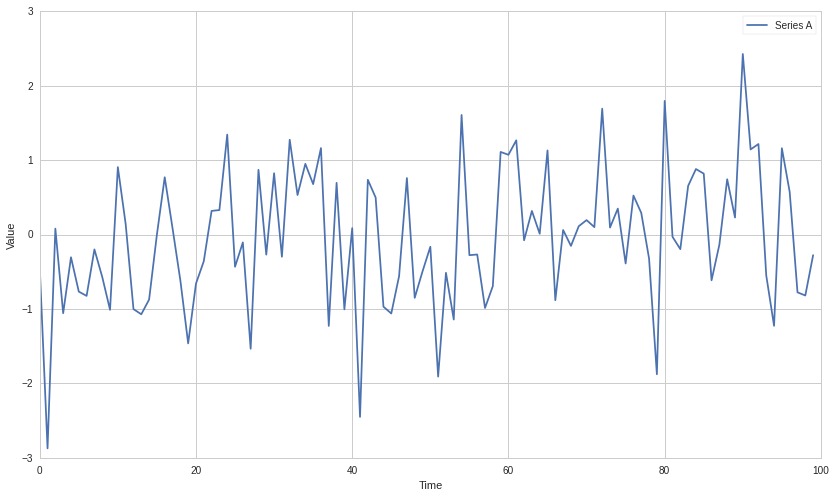### Series B¶

In :
# Set the number of datapoints
T = 100

B = pd.Series(index=range(T))
B.name = 'B'

for t in range(T):
# Now the parameters are dependent on time
# Specifically, the mean of the series changes over time
params = (t * 0.1, 1)
B[t] = generate_datapoint(params)

plt.plot(B)
plt.xlabel('Time')
plt.ylabel('Value')
plt.legend(['Series B']);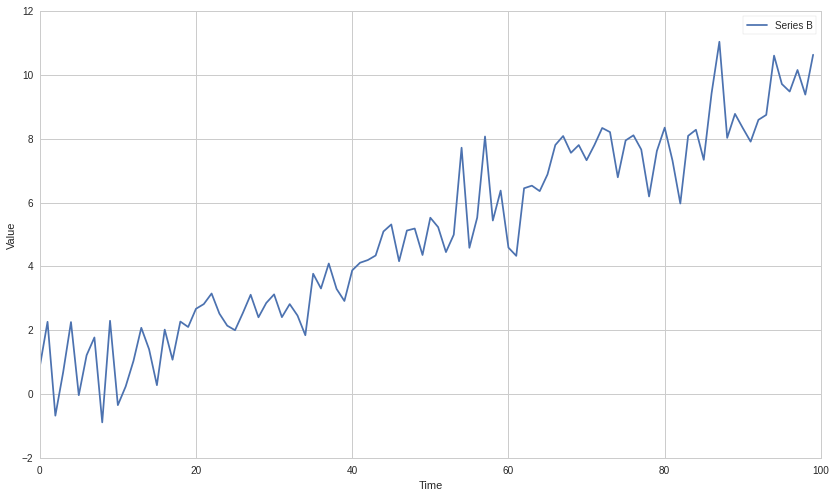### Why Non-Stationarity is Dangerous¶

Many statistical tests, deep down in the fine print of their assumptions, require that the data being tested are stationary. Also, if you naively use certain statistics on a non-stationary data set, you will get garbage results. As an example, let's take an average through our non-stationary $B$.

In :
m = np.mean(B)

plt.plot(B)
plt.hlines(m, 0, len(B), linestyles='dashed', colors='r')
plt.xlabel('Time')
plt.ylabel('Value')
plt.legend(['Series B', 'Mean']);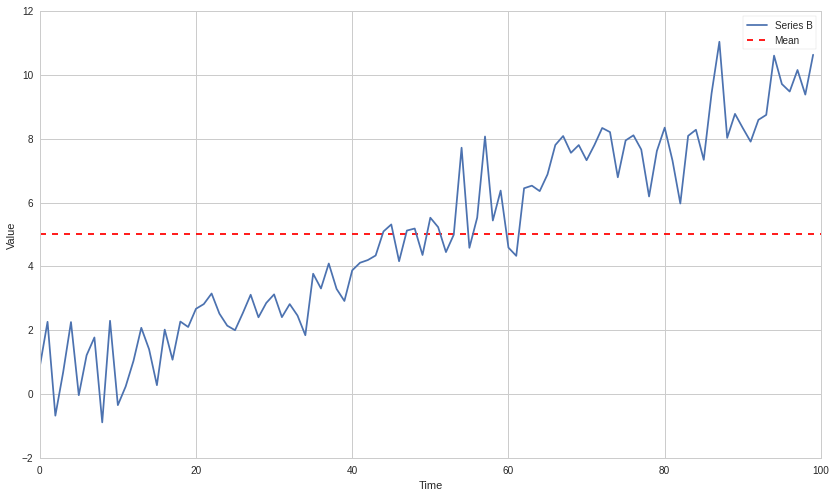The computed mean will show the mean of all data points, but won't be useful for any forecasting of future state. It's meaningless when compared with any specfic time, as it's a collection of different states at different times mashed together. This is just a simple and clear example of why non-stationarity can screw with analysis, much more subtle problems can arise in practice.

### Testing for Stationarity¶

Now we want to check for stationarity using a statistical test.

In :
def check_for_stationarity(X, cutoff=0.01):
# H_0 in adfuller is unit root exists (non-stationary)
# We must observe significant p-value to convince ourselves that the series is stationary
if pvalue < cutoff:
print 'p-value = ' + str(pvalue) + ' The series ' + X.name +' is likely stationary.'
return True
else:
print 'p-value = ' + str(pvalue) + ' The series ' + X.name +' is likely non-stationary.'
return False

In :
check_for_stationarity(A);
check_for_stationarity(B);

p-value = 0.000498500723545 The series A is likely stationary.
p-value = 0.948244716942 The series B is likely non-stationary.


Sure enough, the changing mean of the series makes it non-stationary. Let's try an example that might be a little more subtle.

In :
# Set the number of datapoints
T = 100

C = pd.Series(index=range(T))
C.name = 'C'

for t in range(T):
# Now the parameters are dependent on time
# Specifically, the mean of the series changes over time
params = (np.sin(t), 1)
C[t] = generate_datapoint(params)

plt.plot(C)
plt.xlabel('Time')
plt.ylabel('Value')
plt.legend(['Series C']);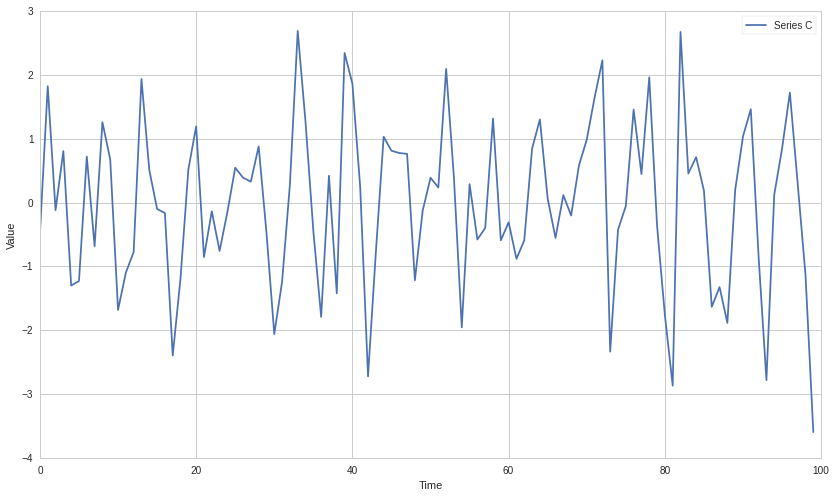A cyclic movement of the mean will be very difficult to tell apart from random noise. In practice on noisy data and limited sample size it can be hard to determine if a series is stationary and whether any drift is random noise or part of a trend. In each individual case the test may or may not pick up subtle effects like this.

In :
check_for_stationarity(C);

p-value = 0.219590266677 The series C is likely non-stationary.


## Order of Integration¶

### Moving Average Representation/Wold's Theorem¶

An important concept in time series analysis is moving average representation. We will discuss this briefly here, but a more complete explanation is available in the AR, MA, and ARMA Models lectures of the Quantopian Lecture Series. Also check Wikipedia as listed below.

This representation expresses any time series $Y_t$ as

$$Y_t = \sum_{j=0}^\infty b_j \epsilon_{t-j} + \eta_t$$
• $\epsilon$ is the 'innovation' series
• $b_j$ are the moving average weights of the innovation series
• $\eta$ is a deterministic series

The key here is as follows. $\eta$ is deterministic, such as a sine wave. Therefore we could perfectly model it. The innovation process is stochastic and there to simulate new information occuring over time. Specifically, $\epsilon_t = \hat Y_t - Y_t$ where $\hat Y_t$ is the in the optimal forecast of $Y_t$ using only information from time before $t$. In other words, the best prediction you can make at time $t-1$ cannot account for the randomness in $\epsilon$.

Each $b_j$ just says how much previous values of $\epsilon$ influence $Y_t$.

### Back to Order of Integration¶

We will note integration order-i as $I(i)$.

A time series is said to be $I(0)$ if the following condition holds in a moving average representation. In hand-wavy english, the autocorrelation of the series decays sufficiently quickly.

$$\sum_{k=0}^\infty |b_k|^2 < \infty$$

This property turns out to be true of all stationary series, but by itself is not enough for stationarity to hold. This means that stationarity implies $I(0)$, but $I(0)$ does not imply stationarity. For more on orders of integration, please see the following links.

### Testing for $I(0)$¶

In practice testing whether the sum of the autocorrelations is finite may not be possible. It is possible in a mathematical derivation, but when we have a finite set of data and a finite number of estimated autocorrelations, the sum will always be finite. Given this difficulty, tests for $I(0)$ rely on stationarity implying the property. If we find that a series is stationary, then it must also be $I(0)$.

Let's take our original stationary series A. Because A is stationary, we know it's also $I(0)$.

In :
plt.plot(A)
plt.xlabel('Time')
plt.ylabel('Value')
plt.legend(['Series A']);### Inductively Building Up Orders of Integration¶

If one takes an $I(0)$ series and cumulatively sums it (discrete integration), the new series will be $I(1)$. Notice how this is related to the calculus concept of integration. The same relation applies in general, to get $I(n)$ take an $I(0)$ series and iteratively take the cumulative sum $n$ times.

Now let's make an $I(1)$ series by taking the cumulative sum of A.

In :
A1 = np.cumsum(A)

plt.plot(A1)
plt.xlabel('Time')
plt.ylabel('Value')
plt.legend(['Series A1']);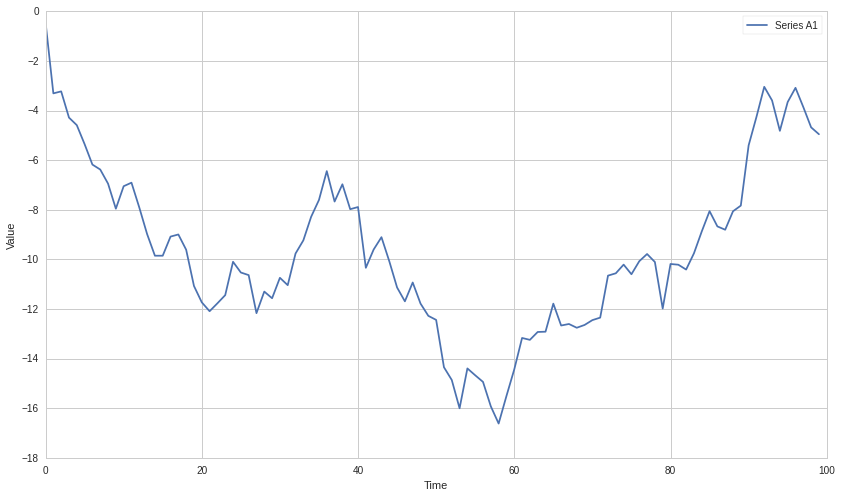Now let's make one $I(2)$ by taking the cumlulative sum again.

In :
A2 = np.cumsum(A1)

plt.plot(A2)
plt.xlabel('Time')
plt.ylabel('Value')
plt.legend(['Series A2']);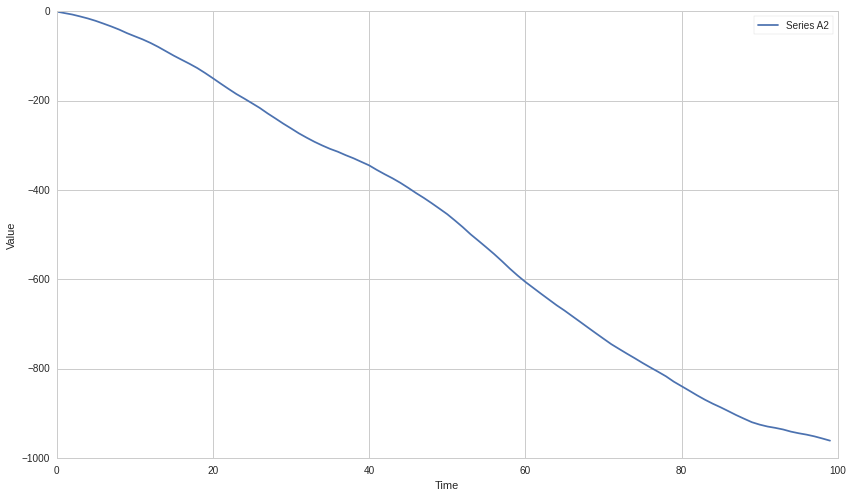### Breaking Down Orders of Integration¶

Conversely, to find the order of integration of a given series, we perform the inverse of a cumulative sum, which is the $\Delta$ or itemwise difference function. Specifically

$$(1-L) X_t = X_t - X_{t-1} = \Delta X$$$$(1-L)^d X_t$$

In this case $L$ is the lag operator. Sometimes also written as $B$ for 'backshift'. $L$ fetches the second to last elements in a time series, and $L^k$ fetches the k-th to last elements. So

$$L X_t = X_{t-1}$$

and

$$(1-L) X_t = X_t - X_{t-1}$$

A series $Y_t$ is $I(1)$ if the $Y_t - Y_t-1$ is $I(0)$. In other words, if you take an $I(0)$ series and cumulatively sum it, you should get an $I(1)$ series.

### Important Take-Away¶

Once all the math has settled, remember that any stationary series is $I(0)$

## Real Data¶

Let's try this out on some real pricing data.

In :
symbol_list = ['MSFT']
prices = get_pricing(symbol_list, fields=['price']
, start_date='2014-01-01', end_date='2015-01-01')['price']
prices.columns = map(lambda x: x.symbol, prices.columns)
X = prices['MSFT']

In :
check_for_stationarity(X);

p-value = 0.666326790934 The series MSFT is likely non-stationary.


Let's take a look, certainly has the warning signs of a non-stationary series.

In :
plt.plot(X.index, X.values)
plt.ylabel('Price')
plt.legend([X.name]);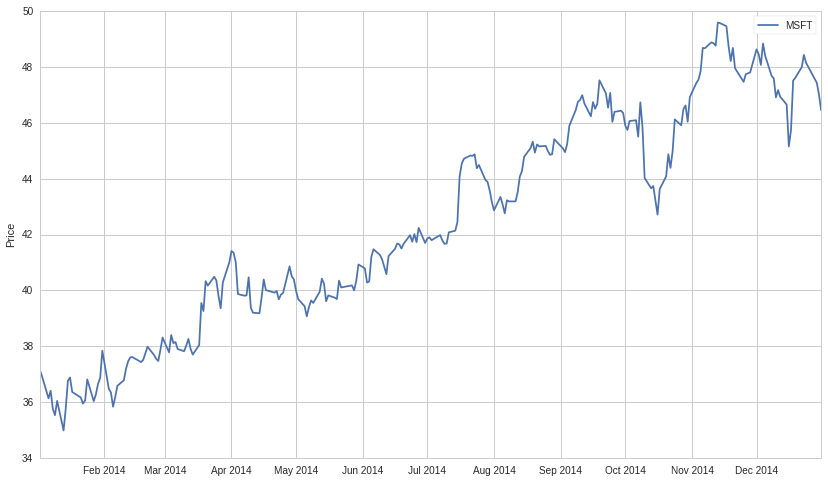Now let's take the delta of the series, giving us the additive returns. We'll check if this is stationary.

In :
X1 = X.diff()[1:]
X1.name = X.name + ' Additive Returns'
check_for_stationarity(X1)
plt.plot(X1.index, X1.values)
plt.legend([X1.name]);

p-value = 1.48184901469e-28 The series MSFT Additive Returns is likely stationary.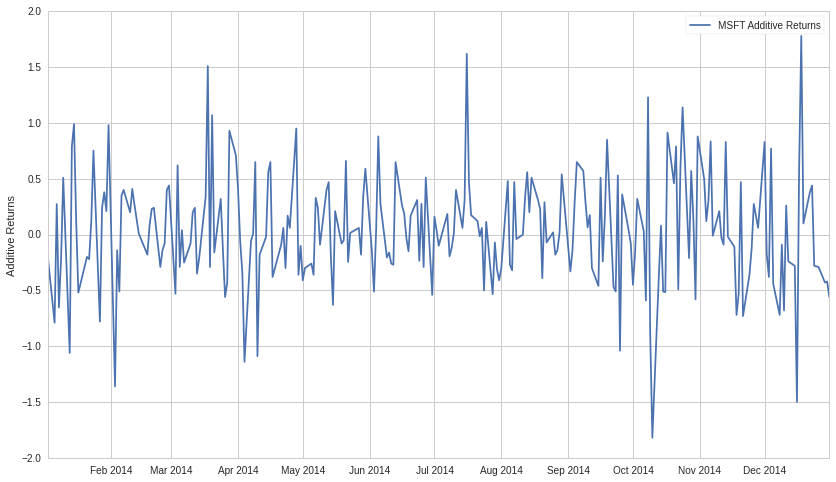Seems like the additive returns are stationary over 2014. That means we will probably be able to model the returns much better than the price. It also means that the price was $I(1)$.

Let's also check the multiplicative returns.

In :
X1 = X.pct_change()[1:]
X1.name = X.name + ' Multiplicative Returns'
check_for_stationarity(X1)
plt.plot(X1.index, X1.values)
plt.ylabel('Multiplicative Returns')
plt.legend([X1.name]);

p-value = 8.05657888734e-29 The series MSFT Multiplicative Returns is likely stationary.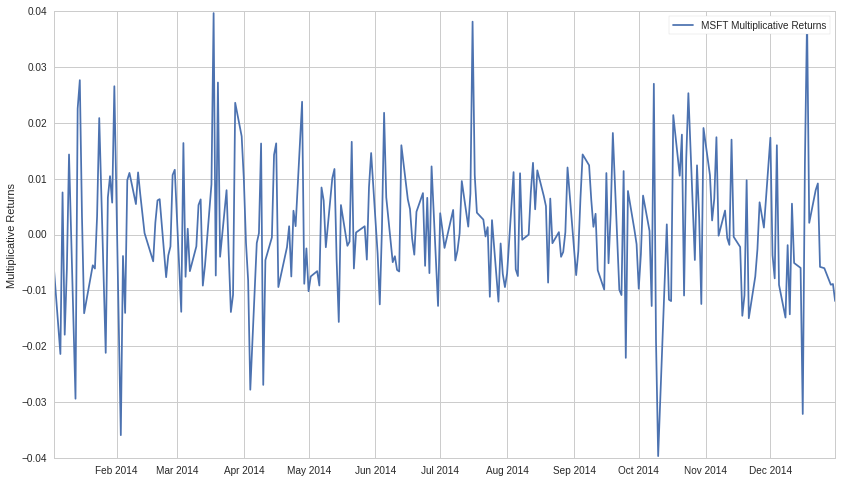Seems like the multiplicative returns are also stationary. Both the multiplicative and additive deltas on a series get at similar pieces of information, so it's not surprising both are stationary. In practice this might not always be the case.

## IMPORTANT NOTE¶

As always, you should not naively assume that because a time series is stationary in the past it will continue to be stationary in the future. Tests for consistency of stationarity such as cross validation and out of sample testing are necessary. This is true of any statistical property, we just reiterate it here. Returns may also go in and out of stationarity, and may be stationary or non-stationary depending on the timeframe and sampling frequency.

## Note: Returns Analysis¶

The reason returns are usually used for modeling in quantitive finance is that they are far more stationary than prices. This makes them easier to model and returns forecasting more feasible. Forecasting prices is more difficult, as there are many trends induced by their $I(1)$ integration. Even using a returns forecasting model to forecast price can be tricky, as any error in the returns forecast will be magnified over time.

## Cointegration¶

Finally, now that we've discussed stationarity and order of integration, we can discuss cointegration.

### Def: Linear Combination¶

A linear combination of the time series ($X_1$, $X_2$, $\dots$, $X_k$) is a new time series $Y$ constructed as follows for any set of real numbers $b_1 \dots b_k$

$$Y = b_1X_1 + b_2X_2 + \dots + b_kX_k$$

### Formal Definition¶

The formal definition of cointegration is as follows.

For some set of time series ($X_1$, $X_2$, $\dots$, $X_k$), if all series are $I(1)$, and some linear combination of them is $I(0)$, we say the set of time series is cointegrated.

#### Example¶

$X_1$, $X_2$, and $X_3$ are all $I(1)$, and $2X_1 + X_2 + 0X_3 = 2X_1 + X_2$ is $I(0)$. In this case the time series are cointegrated.

### Intuition¶

The intuition here is that for some linear combination of the series, the result lacks much auto-covariance and is mostly noise. This is useful for cases such as pairs trading, in which we find two assets whose prices are cointegrated. Since the linear combination of their prices $b_1A_1 + b_2A_2$ is noise, we can bet on the relationship $b_1A_1 + b_2A_2$ mean reverting and place trades accordingly. See the Pairs Trading Lecture in the Quantopian Lecture Series for more information.

### Simulated Data Example¶

Let's make some data to demonstrate this.

In :
# Length of series
N = 100

# Generate a stationary random X1
X1 = np.random.normal(0, 1, N)
# Integrate it to make it I(1)
X1 = np.cumsum(X1)
X1 = pd.Series(X1)
X1.name = 'X1'

# Make an X2 that is X1 plus some noise
X2 = X1 + np.random.normal(0, 1, N)
X2.name = 'X2'

In :
plt.plot(X1)
plt.plot(X2)
plt.xlabel('Time')
plt.ylabel('Series Value')
plt.legend([X1.name, X2.name]);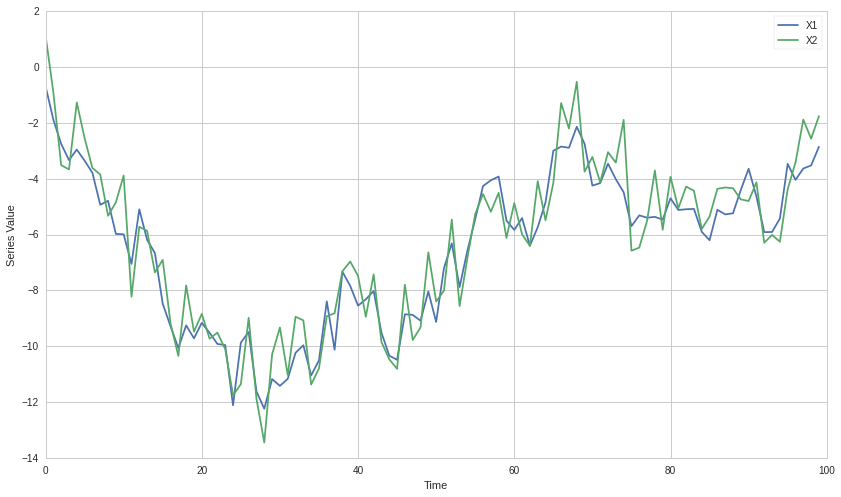Because $X_2$ is just an $I(1)$ series plus some stationary noise, it should still be $I(1)$. Let's check this.

In :
Z = X2.diff()[1:]
Z.name = 'Z'

check_for_stationarity(Z);

p-value = 3.06566830522e-19 The series Z is likely stationary.


Looks good. Now to show cointegration we'll need to find some linear combination of $X_1$ and $X_2$ that is stationary. We can take $X_2-X_1$. All that's left over should be stationary noise by design. Let's check this.

In :
Z = X2 - X1
Z.name = 'Z'

plt.plot(Z)
plt.xlabel('Time')
plt.ylabel('Series Value')
plt.legend(['Z']);

check_for_stationarity(Z);

p-value = 1.03822288113e-18 The series Z is likely stationary.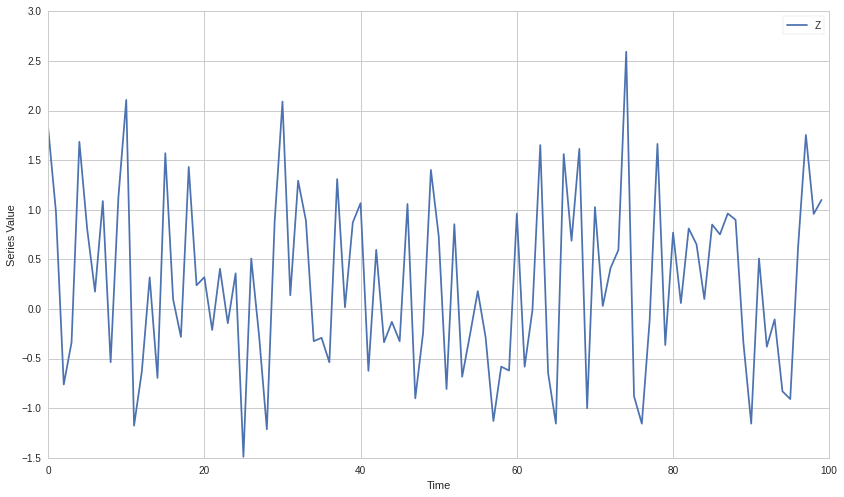### Testing for Cointegration¶

There are a bunch of ways to test for cointegration. This wikipedia article describes some. In general we're just trying to solve for the coefficients $b_1, \dots b_k$ that will produce an $I(0)$ linear combination. If our best guess for these coefficients does not pass a stationarity check, then we reject the hypothesis that the set is cointegrated. This will lead to risk of Type II errors (false negatives), as we will not exhaustively test for stationarity on all coefficent combinations. However Type II errors are generally okay here, as they are safe and do not lead to us making any wrong forecasts.

In practice a common way to do this for pairs of time series is to use linear regression to estimate $\beta$ in the following model.

$$X_2 = \alpha + \beta X_1 + \epsilon$$

The idea is that if the two are cointegrated we can remove $X_2$'s depedency on $X_1$, leaving behind stationary noise. The combination $X_2 - \beta X_1 = \alpha + \epsilon$ should be stationary.

#### Real Data Example¶

Let's try on some real data. We'll get prices and plot them first.

In :
symbol_list = ['ABGB', 'FSLR']
prices = get_pricing(symbol_list, fields=['price']
, start_date='2014-01-01', end_date='2015-01-01')['price']
prices.columns = map(lambda x: x.symbol, prices.columns)
X1 = prices[symbol_list]
X2 = prices[symbol_list]

In :
plt.plot(X1.index, X1.values)
plt.plot(X1.index, X2.values)
plt.xlabel('Time')
plt.ylabel('Series Value')
plt.legend([X1.name, X2.name]);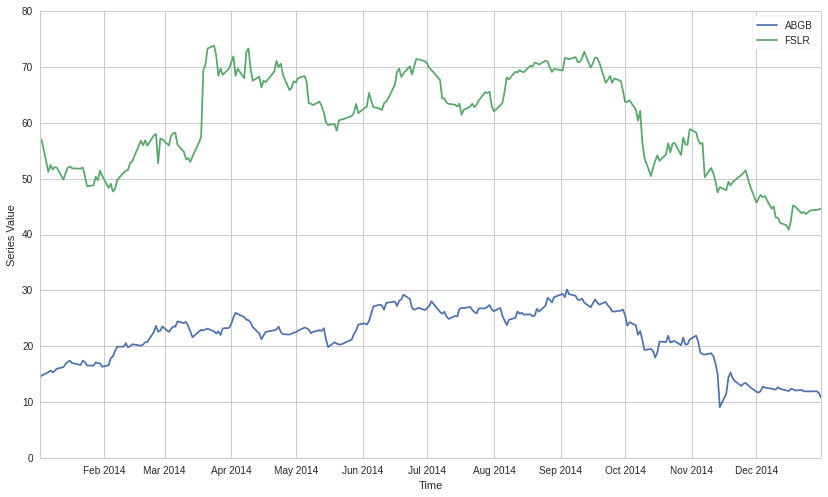Now use linear regression to compute $\beta$.

In :
X1 = sm.add_constant(X1)
results = sm.OLS(X2, X1).fit()

# Get rid of the constant column
X1 = X1[symbol_list]

results.params

Out:
const    26.609769
ABGB      1.536686
dtype: float64
In :
b = results.params[symbol_list]
Z = X2 - b * X1
Z.name = 'Z'

plt.plot(Z.index, Z.values)
plt.xlabel('Time')
plt.ylabel('Series Value')
plt.legend([Z.name]);

check_for_stationarity(Z);

p-value = 0.000972948552814 The series Z is likely stationary.